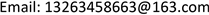﻿ 别墅屋顶并网光伏发电系统投资效益分析 Analysis on Investment Benefit of Roof and Grid Photovoltaic Power Generation System of Villa

Journal of Low Carbon Economy
Vol.06 No.04(2017), Article ID:22728,9 pages
10.12677/JLCE.2017.64006

Analysis on Investment Benefit of Roof and Grid Photovoltaic Power Generation System of Villa

Xingang Zhao, Jie Chen

School of Economics and Management, North China Electric Power University, BeijingReceived: Oct. 29th, 2017; accepted: Nov. 12th, 2017; published: Nov. 20th, 2017ABSTRACT

PV building integration is one of the effective means to solve the high energy consumption of China's building, but it is not popular in the residential building of our country. The core problem is that the investment benefit of PV project is not high. Therefore, this paper takes the villa users as the research object, and studies the investment benefit of the roof grid-connected PV system under different influence on the Internet mode, electricity consumption and subsidy policy. It is suggested that the government implements different subsidy policies based on electricity consumption, gradually cancels cross subsidies and carries out the peak-valley time-of-use tariff policy.

Keywords:BAPV, Investment Benefit, Peak-Valley Time-of-Use Tariff1. 引言

2. 别墅用户光伏建筑投资效益分析模型

2.1. 成本分析

1) 初始投资成本，即太阳能电池组件、支架、逆变器、电缆以及系统安装成本等的总和；

2) 经常性运营成本，即光伏发电系统的定期清洁维护成本，与系统的装机规模有关，一般为50元/(KW·年)  ；

3) 财务费用，主要是借款的利息费用，采取分期偿还、等额本息的方式还款，可以用下式表示：

${C}_{n}={C}_{0}{X}_{1}R$

2.2. 收益分析

1) 全额上网

${I}_{d}^{n}={E}_{d}^{n}{P}_{C1}$

2) 自发自用，余电上网

1) 日发电量大于白天用电量时，业主收益：

${I}_{d}^{n}={E}_{d}^{n}\left({G}_{p1}+{G}_{p2}\right)+\left({E}_{d}^{n}-{Q}_{d}^{n}{X}_{3}\right){P}_{C2}+{Q}_{d}^{n}{P}_{C3}-{Q}_{d}^{n}\left(1-{X}_{3}\right){P}_{C3}$

2) 日发电量小于白天用电量时，业主收益：

${I}_{d}^{n}={E}_{d}^{n}\left({G}_{P1}+{G}_{P2}\right)+{Q}_{d}^{n}{P}_{C3}-\left({Q}_{d}^{n}-{E}_{d}^{n}\right){P}_{C3}$

2.3. 光伏建筑投资效益评估模型

2.3.1. 平准化度电成本

$LCOE=\frac{{C}_{0}+\underset{n=1}{\overset{N}{\sum }}\frac{{I}_{n}}{{\left(1+{i}_{0}\right)}^{n}}}{\underset{n=1}{\overset{N}{\sum }}\frac{{E}_{n}}{{\left(1+{i}_{0}\right)}^{n}}}$

2.3.2. 净现值

$NPV=\underset{n=0}{\overset{N}{\sum }}{\left(CI-CO\right)}_{n}{\left(1+{i}_{0}\right)}^{-n}$

1) 若 $NPV\ge 0$ ，则项目应予接受；

2) 若 $NPV<0$ ，则项目应拒绝。

2.3.3. 内部收益率

$NPV\left(IRR\right)=\underset{n=0}{\overset{N}{\sum }}{\left(CI-CO\right)}_{n}{\left(1+IRR\right)}^{-n}=0$

1) 若 $IRR\ge {i}_{0}$ ，则项目在经济效果上可以接受；

2) 若 $IRR<{i}_{0}$ ，则项目在经济效果上不可接受。

2.3.4. 投资回收期

$\underset{n=0}{\overset{{T}_{P}}{\sum }}\left(C{I}_{n}-C{O}_{n}\right)=K$

2.3.5. 净现值指数

$NPVI=\frac{\underset{n=0}{\overset{N}{\sum }}{\left(CI-CO\right)}_{n}{\left(1+{i}_{0}\right)}^{-n}}{\underset{n=0}{\overset{N}{\sum }}{K}_{n}{\left(1+{i}_{0}\right)}^{-n}}$

3. 别墅案例分析

3.1. 案例项目简介

3.2. 项目的成本分析

1) 初始投资成本12748.92元/KW，即总初始投资成本为38144.76元；

2) 经常性运营成本为149.6元/年。

3.3. 项目的发电量分析

3.4. 项目的用电量分析

3.5. 项目的投资效益分析

1) “全额上网”模式

“全额上网”方式下，项目的净现值小于0，资金的利用率比较低，因此项目不可行，本项目的平准化度电成本为1.16元/KWh，而光伏上网标杆电价为0.85元/KWh，小于成本，所以“全额上网”的经济效益并不理想。

2) “自发自用，余电上网”模式

“自发自用，余电上网”模式下净现值大于0，项目可行，资金的利用效率比较高，投资回收期也比较合理。

① 不同地方补贴

② 不同用电量Table 2. The investment efficiency of case project under full internet mode

③ 峰谷阶梯电价

④ 售电侧开放Table 3. The investment benefit of case project under self-use modeTable 4. The effect of the decline of local subsidy on net present value in self-use mode, yuanTable 5. Benefit of project investment under different electricity consumptionTable 6. Investment benefit of project under the system of peak and valleyTable 7. Investment benefit of different residents’ electricity price

4. 政策建议

1) 尽早解决交叉补贴问题

2) 推广峰谷阶梯电价

3) 鼓励别墅等有条件的用户率先安装光伏

5. 结论与展望

Analysis on Investment Benefit of Roof and Grid Photovoltaic Power Generation System of Villa[J]. 低碳经济, 2017, 06(04): 37-45. http://dx.doi.org/10.12677/JLCE.2017.64006

1. 1. 马文生, 郝斌. 光伏建筑一体化相关问题的探讨[J]. 可再生能源, 2011(29): 94-97.

2. 2. 张鸣, 蔡亮, 虞维平. BIPV系统经济性分析[J]. 应用能源技术, 2007(11): 1-4.

3. 3. 吕双辉, 蔡声霞, 王守相. 分布式光伏–储能系统的经济性评估及发展建议[J]. 中国电力, 2015(48): 139-144.

4. 4. 邵汉桥, 张籍, 张维. 分布式光伏发电经济性及政策分析[J]. 电力建设, 2014(35): 51-57.

5. 5. Seng, L.Y., Lalchand, G. and Lin, G.M.S. (2008) Economical, Environmental and Technical Analysis of Building Inte-grated Photovoltaic Systems in Malaysia. Energy Policy, 36, 2130-2142. https://doi.org/10.1016/j.enpol.2008.02.016

6. 6. Bernal-Agustín, J.L. and Dufo-López, R. (2006) Economical and Environmental Analysis of Grid Connected Photovoltaic Systems in Spain. Renewable Energy, 31, 1107-1128. https://doi.org/10.1016/j.renene.2005.06.004

7. 7. Ren, H., Gao, W. and Ruan, Y. (2009) Economic Optimization and Sensitivity Analysis of Photovoltaic System in Residential Buildings. Renewable Energy, 34, 883-889. https://doi.org/10.1016/j.renene.2008.06.011

8. 8. 沙浩洁, 李永光, 杨天海. 结合别墅用户的分布式风力发电经济性评估[J]. 上海电力, 2009(1): 42-44.